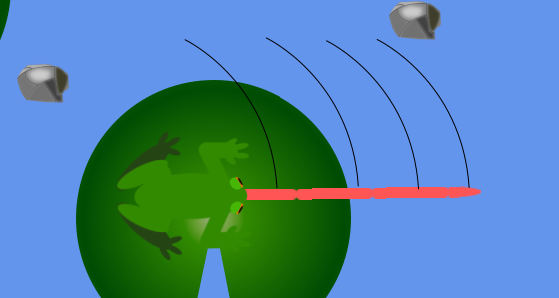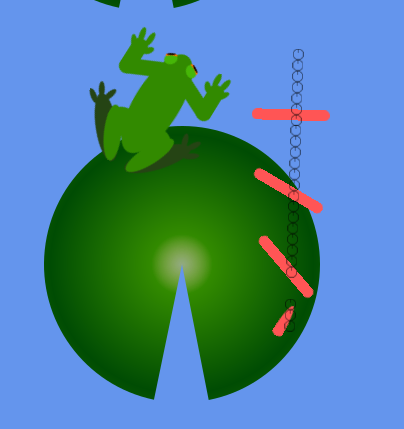# Off-axis vector 2 rotation

I’m trying to rotate a bunch of Vector2s that are off-axis.

The tongue is comprised of 4 sections and I would like them to rotate with the frog.I know this can be calculated with:
x’ = xcos(angle) - ysin(angle)
y’ = xsin(angle) + ycos(angle)

`Position += new Vector2(Tongue.DEV_MOMENTUM.X * (float)(Math.Cos(Tongue.DEV_ROTATION) - Tongue.DEV_MOMENTUM.Y * (float)(Math.Sin(Tongue.DEV_ROTATION))), Tongue.DEV_MOMENTUM.X * (float)(Math.Sin(Tongue.DEV_ROTATION) + Tongue.DEV_MOMENTUM.Y * (float)(Math.Cos(Tongue.DEV_ROTATION))));`

in the above example Tongue.DEV_MOMENTUM = (1,1).

But the result I get is that each piece rotates around some invisible axis that is nowhere close to what I want.
Result:If you need any more info just ask, I spent to much time on this and have the feeling that I’m the stupidest member of Monogame communityIt looks like you want to rotate each piece around a pivot point, which is the frog. You can do that with the following method:

``````/// <summary>
/// Rotates a Vector2 around another Vector2 representing a pivot.
/// </summary>
/// <param name="vector">The Vector2 to rotate.</param>
/// <param name="pivot">A Vector2 representing the pivot to rotate around.</param>
/// <param name="angle">The rotation angle, in radians.</param>
/// <returns>A Vector2 rotated around <paramref name="pivot"/> by <paramref name="angle"/>.</returns>
public static Vector2 RotateVectorAround(Vector2 vector, Vector2 pivot, double angle)
{
//Get the X and Y difference
float xDiff = vector.X - pivot.X;
float yDiff = vector.Y - pivot.Y;

//Rotate the vector
float x = (float)((Math.Cos(angle) * xDiff) - (Math.Sin(angle) * yDiff) + pivot.X);
float y = (float)((Math.Sin(angle) * xDiff) + (Math.Cos(angle) * yDiff) + pivot.Y);

return new Vector2(x, y);
}
``````

You can use the frog’s rotation for each section of the tongue.

Definitely not; don’t be afraid to ask questions!

2 Likes

It looks like you got the brackets wrong. Instead of this:

x’ = xcos(angle) - ysin(angle)

you got this:

x’ = x*(cos(angle) - y*sin(angle))

1 Like

Of course, the pivot, I forgot to include it into my method goddammit, thank you so very much friend.